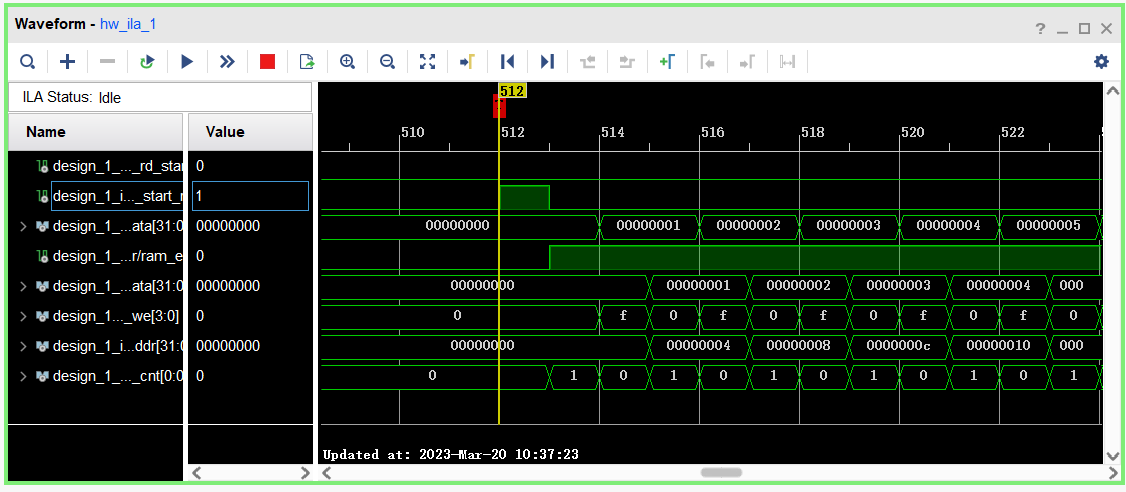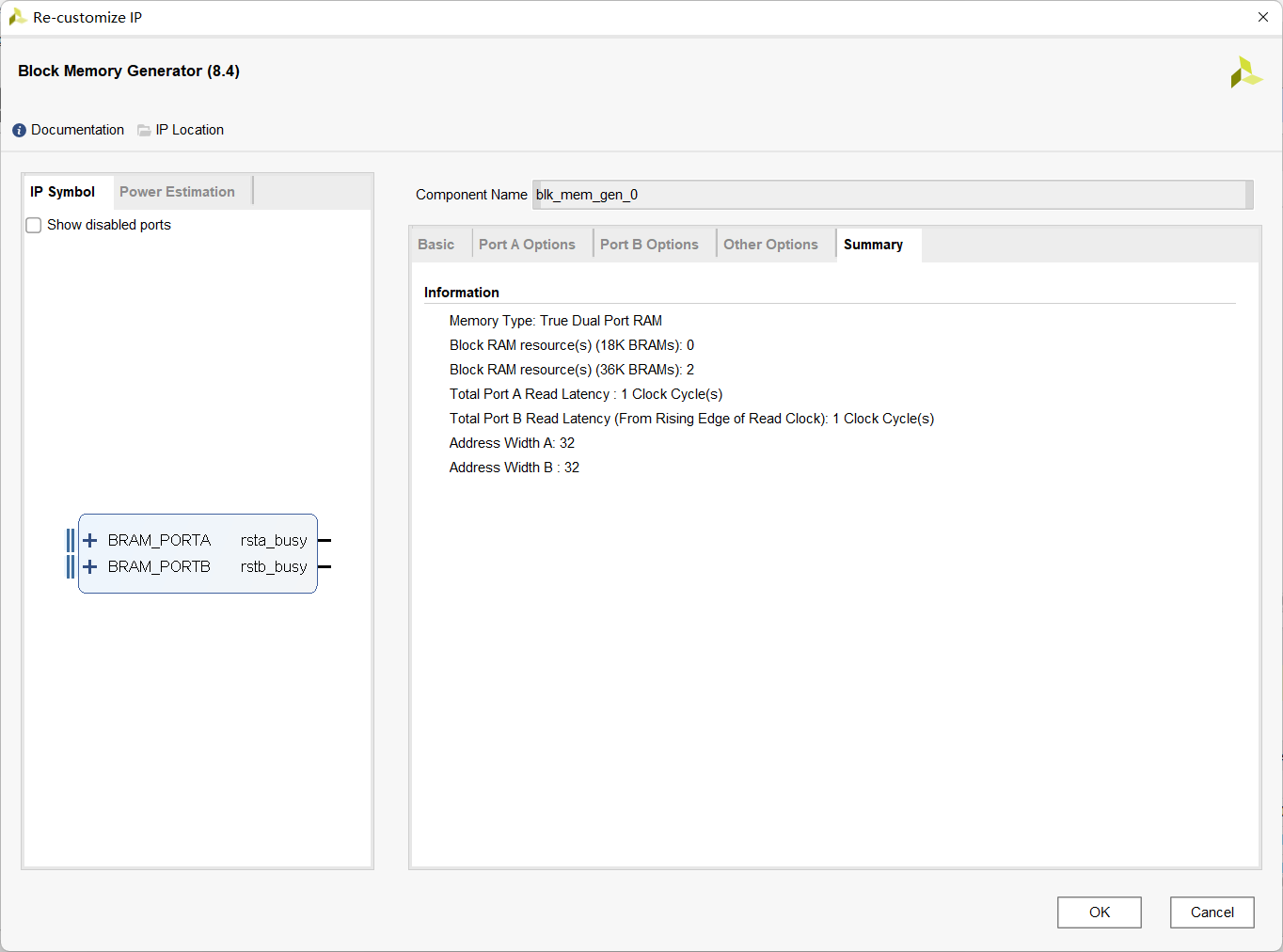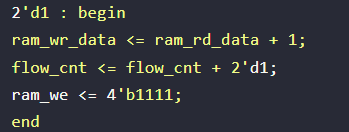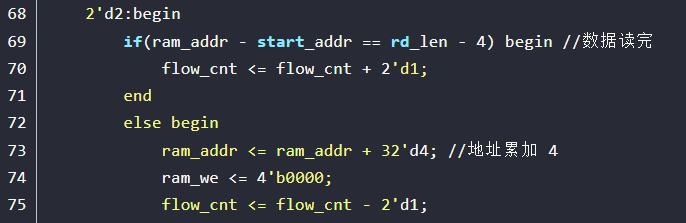# 使用BRAM进行PL与PS通信及ILA使用实例

## IP核内容处理

``````output wire ram_clk ,                //RAM 时钟
input wire [31:0] ram_rd_data,       //RAM 中读出的数据
output wire ram_en ,                 //RAM 使能信号
output wire [31:0] ram_addr ,        //RAM 地址
output wire [3:0] ram_we ,           //RAM 读写控制信号
output wire [31:0] ram_wr_data,      //RAM 写数据
output wire ram_rst ,                //RAM 复位信号,高电平有``````

``````.ram_clk (ram_clk ),
.ram_rd_data (ram_rd_data),
.ram_en (ram_en ),
.ram_we (ram_we ),
.ram_wr_data (ram_wr_data),
.ram_rst (ram_rst )``````

``````bram_wr inst_bram_wr(
.clk (S_AXI_ACLK),
.rst_n (S_AXI_ARESETN),
.start_rd (slv_reg0),
.rd_len (slv_reg2),
.pl_wr_done (pl_wr_done),//用于传递PL操作完成标识
.ps_rd_start (slv_reg4),//用于传递PS开始读取标识
//RAM 端口
.ram_clk (ram_clk ),
.ram_rd_data (ram_rd_data),
.ram_en (ram_en ),
.ram_we (ram_we ),
.ram_wr_data (ram_wr_data),
.ram_rst (ram_rst )
);``````

``````output wire ram_clk ,                //RAM 时钟
input wire [31:0] ram_rd_data,       //RAM 中读出的数据
output wire ram_en ,                 //RAM 使能信号
output wire [31:0] ram_addr ,        //RAM 地址
output wire [3:0] ram_we ,           //RAM 读写控制信号
output wire [31:0] ram_wr_data,      //RAM 写数据
output wire ram_rst ,                //RAM 复位信号,高电平有``````

``````module bram_wr(
input clk , //时钟信号
input rst_n , //复位信号
input start_rd , //读开始信号
input [31:0] rd_len , //读数据的长度
output reg pl_wr_done ,    //pl端写出完毕
input ps_rd_start ,    //ps端开始读取
//RAM 端口
output ram_clk , //RAM 时钟
input [31:0] ram_rd_data, //RAM 中读出的数据
output reg ram_en , //RAM 使能信号
output reg [31:0] ram_addr , //RAM 地址
output reg [3:0] ram_we , //RAM 读写控制信号
output reg [31:0] ram_wr_data, //RAM 写数据
output ram_rst //RAM 复位信号,高电平有效
);

//reg define
reg [2:0] flow_cnt;
reg start_rd_d0;
reg start_rd_d1;
//wire define

//*****************************************************
//** main code
//*****************************************************

assign ram_rst = 1'b0;
assign ram_clk = clk ;         //时钟clk
assign pos_start_rd = ~start_rd_d1 & start_rd_d0;  //PL端操作开始信号

//延时两拍，采 start_rd 信号的上升沿
always @(posedge clk or negedge rst_n) begin
if(!rst_n) begin
start_rd_d0 <= 1'b0;
start_rd_d1 <= 1'b0;
end
else begin
start_rd_d0 <= start_rd;
start_rd_d1 <= start_rd_d0;
end
end

//根据读开始信号,从 RAM 中读出数据
always @(posedge clk or negedge rst_n) begin
if(!rst_n) begin
flow_cnt <= 3'd0;
ram_en <= 1'b0;
ram_we <= 4'd0;
end
else begin
case(flow_cnt)
3'd0 : begin
if(ps_rd_start) begin
flow_cnt <= 3'd0;   //正在被ps端占用
end
else if(pos_start_rd) begin
ram_en <= 1'b1;
flow_cnt <= flow_cnt + 3'd1;
end
end
3'd1 : begin
flow_cnt <= flow_cnt + 3'd1;    //延迟一个时钟周期，原代码的修复一
end
3'd2 : begin
ram_we <= 4'b1111;
ram_wr_data <= ram_rd_data + 32'd1;    //内容加一并写回
flow_cnt <= flow_cnt + 3'd1;
end
3'd3:begin
flow_cnt <= flow_cnt + 3'd1;
ram_we <= 4'b0000;
end
else begin
ram_we <= 4'b0000;      //BRAM写入信号拉低，原代码的修复二
flow_cnt <= flow_cnt - 3'd2;
end
end
3'd4 : begin           //完成状态
flow_cnt <= 3'd5;
ram_en <= 1'b0;      //取消bram使能
pl_wr_done <= 1'b1;  //PL端完成标识
end
3'd5 : begin           //传递PL端完成标识
if (ps_rd_start) begin     //PS端开始读取，完成PL端所有操作
flow_cnt <= 3'd0;
pl_wr_done <= 1'b0;
end
else begin
flow_cnt <= 3'd5;      //PS端开始读取，PL端所有操作完成
end
end
endcase
end
end
endmodule
``````

## SDK部分

``````#include "xil_printf.h"
#include "stdio.h"
#include "bram_wr.h"
#include "xbram.h"
#include "xparameters.h"

#define PL_BRAM_START BRAM_WR_S00_AXI_SLV_REG0_OFFSET //RAM 读开始寄存器信号
#define PL_BRAM_LEN BRAM_WR_S00_AXI_SLV_REG2_OFFSET //PL 读 RAM 的深度

#define START_ADDR 0 //RAM 起始地址 范围:0~1023
#define BRAM_DATA_BYTE 4 //BRAM 数据字节个数

int data; //写入 BRAM 的字符数组
int data_len; //写入 BRAM 的字符个数

//main 函数
int main()
{
int i=0,wr_cnt = 0,k=0;
for(int k=0;k<1024;k++)
{
data[k] = k;
}
data_len = 1024;
//将用户输入的字符串写入 BRAM 中
getchar();//方便调试
//每次循环向 BRAM 中写入 1 个整数（4字节宽度）
for(i = BRAM_DATA_BYTE*START_ADDR ; i < BRAM_DATA_BYTE*(START_ADDR + data_len) ;i += BRAM_DATA_BYTE)
{
wr_cnt++;
}
//设置 BRAM 写入的字符串长度
BRAM_WR_mWriteReg(PL_BRAM_BASE, PL_BRAM_LEN , BRAM_DATA_BYTE*data_len);
//设置 BRAM 的起始地址
//拉高 BRAM 开始信号
BRAM_WR_mWriteReg(PL_BRAM_BASE, PL_BRAM_START , 1);
//拉低 BRAM 开始信号
BRAM_WR_mWriteReg(PL_BRAM_BASE, PL_BRAM_START , 0);
//从 BRAM 中读出数据

//检查可读状态
}

i = 0;
printf("done");//可读
//ps读开始
BRAM_WR_mWriteReg(PL_BRAM_BASE, 16 , 1);
//拉低信号，pl端恢复状态
BRAM_WR_mWriteReg(PL_BRAM_BASE, 16 , 0);

//循环从 BRAM 中读出数据
for(i = BRAM_DATA_BYTE*START_ADDR ; i < BRAM_DATA_BYTE*(START_ADDR + data_len) ;i += BRAM_DATA_BYTE)
{
}
}``````

## 效果演示

PS端向BRAM中写入一个0-1023的递增数列，PL端遍历BRAM，将读入的数据加一并写回，得到以下结果。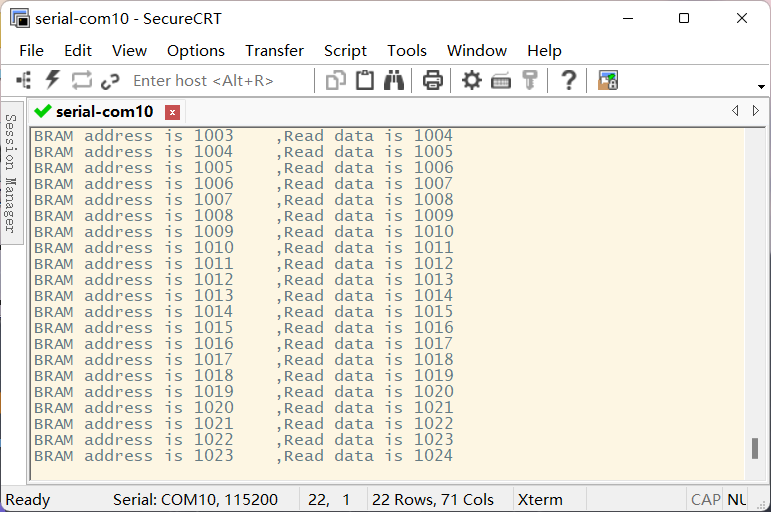## ILA使用及原博主代码错误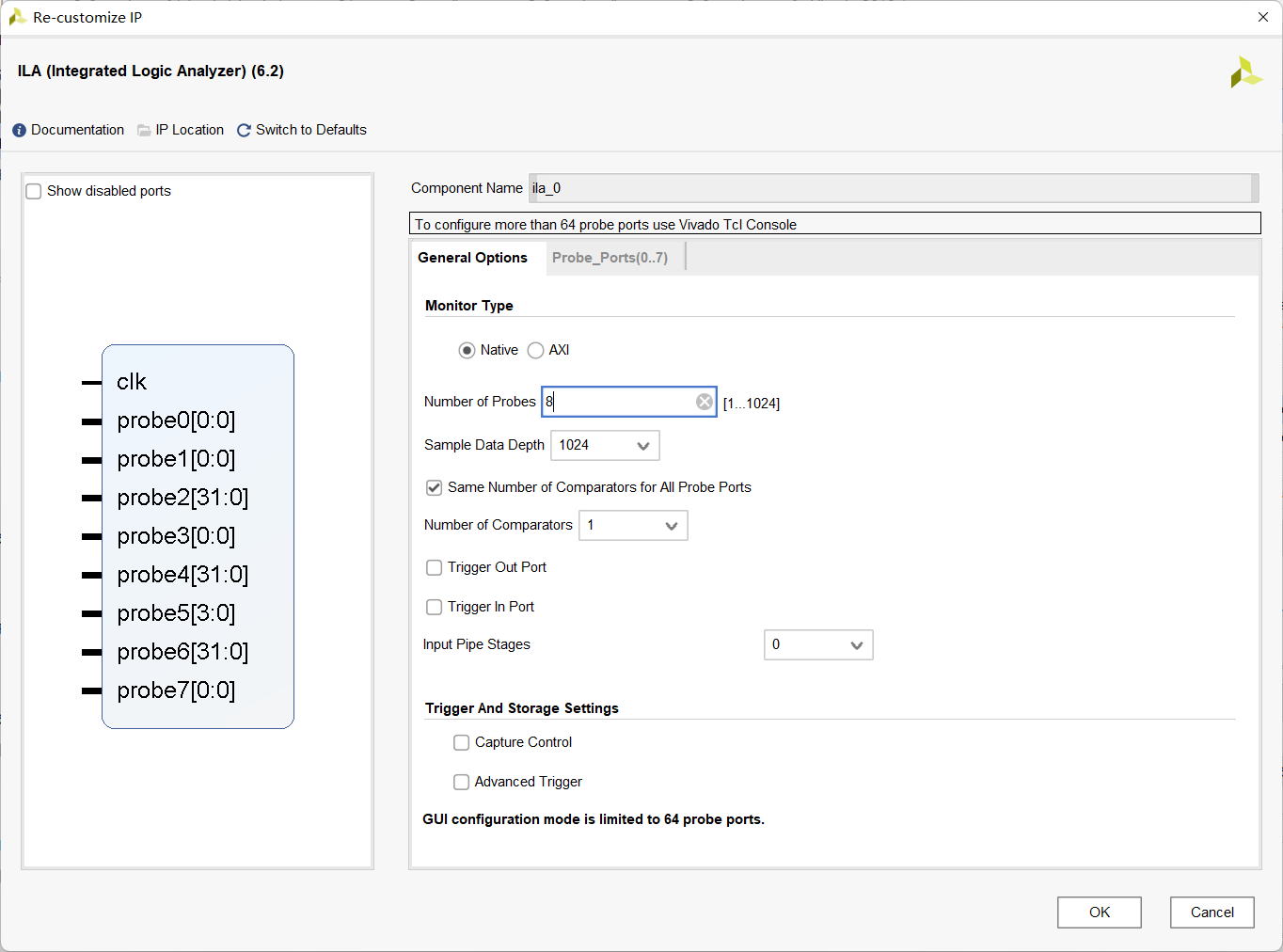``````ila_0 ila_inst (//ila部分，用于DEBUG，不调试可忽略这部分
.clk(ram_clk),          //时钟
.probe0(ps_rd_start),   //ps端开始读取标志
.probe1(pos_start_rd),  //pl端开始操作
.probe2(ram_wr_data),   //pl端写入bram数据
.probe3(ram_en),        //bram enable
.probe4(ram_rd_data),   //pl端读bram数据
.probe5(ram_we),        //bram write enable
.probe7(flow_cnt)       //状态机
);``````